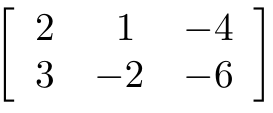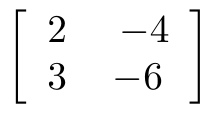# Cramer’s Rule

Cramer’s rule is a method for solving a system of equations using the determinants. In order to do this, you must be familiar with matrices and row operations. Generally, it is really difficult to explain that is a simple matter but there are two main parts to completing thisPart 1:

• Evaluate the determinants using the coefficients aka D
• Evaluate the determinants using the constants in place of x aka Dx
• Evaluate the determinant using the constants in place of y aka Dy

Part 2:

• Find x by  Dx / D
• Find y by Dy / D

This is modified if the system is 3 variables. Below we will go through an example with 2 variables.

Example

Here is our problem

## 2x + y = -4 3x – 2y = -6

Below is the matrix of the system of equationsWe will first evaluate the Determinant D using the coefficients. In other words, we are going to calculate the determinant for the first two columns of the matrix. Below is the answer.What we have just done we will do two more times. Once two find the determinant of x and once to find the determinant of y. When we say determinant x or y we are excluding that column from the 2×2 matrix. In other words, if I want to find the determinant of x I would exclude the x values from the 2×2 matrix when calculating. Below is the determinant of x.Lastly, here is the determinant of yWe no have all the information we need to solve for x and y. To find the answers we do the following

• Dx / D = x
• Dy / D = y

We know these value already so we plug them in as shown below.You can plug in these values into the original equation for verification.

The steps we took here can also be applied to a 3 variable system of equation. In such a situation you would solve one additional determinant for z.

Conclusion

Solving a system of equations using Cramer’s rule is much faster and efficient than other methods. It also requires some additional knowledge of rows and matrices but the benefits far outweigh the challenge of learning some basic rules of row operations.

## 2 thoughts on “Cramer’s Rule”

1.Michael Paul Goldenberg

Looks so much like “Cramer’s Rule”!

2.Randy Keck

Wish I knew this method when I was teaching.